• Slides: 11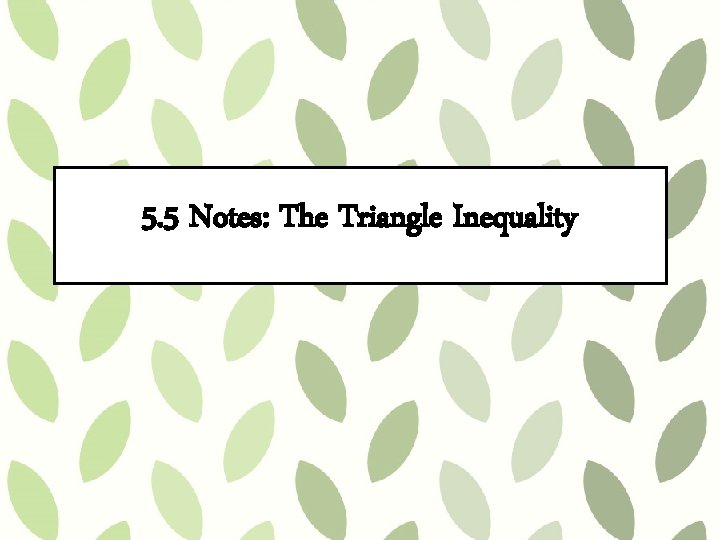5. 5 Notes: The Triangle InequalityVocabulary Triangle Inequality Theorem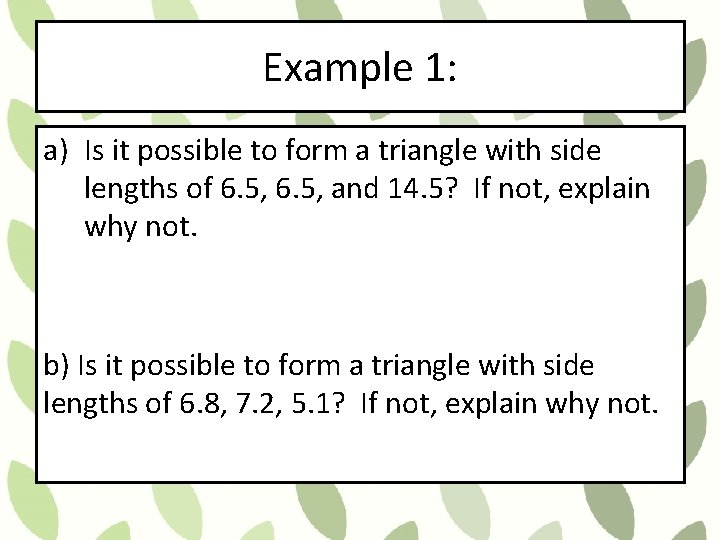Example 1: a) Is it possible to form a triangle with side lengths of 6. 5, and 14. 5? If not, explain why not. b) Is it possible to form a triangle with side lengths of 6. 8, 7. 2, 5. 1? If not, explain why not.Example 2: In ΔPQR, PQ = 7. 2 and QR = 5. 2. Which measure cannot be PR? a) 7 b) 9 c) 11 d) 13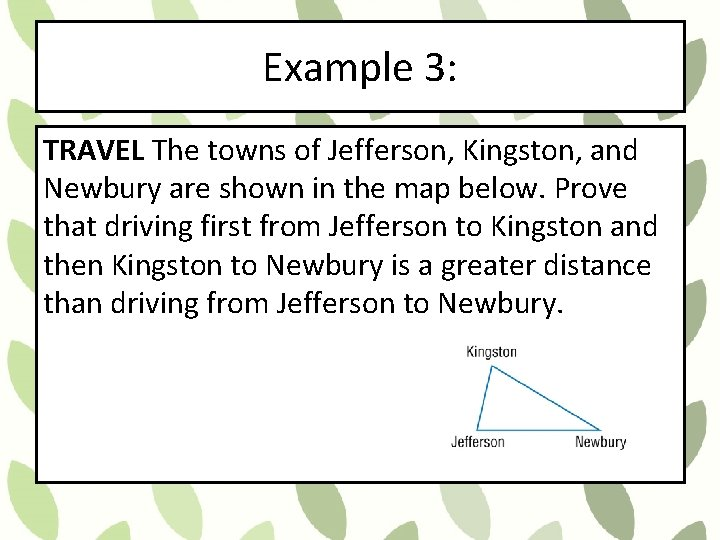Example 3: TRAVEL The towns of Jefferson, Kingston, and Newbury are shown in the map below. Prove that driving first from Jefferson to Kingston and then Kingston to Newbury is a greater distance than driving from Jefferson to Newbury.Example 4: The lengths of two sides of a triangle are given. Find the range of possible lengths for the third side. a. 4, 8 b. 13, 8 c. 10, 15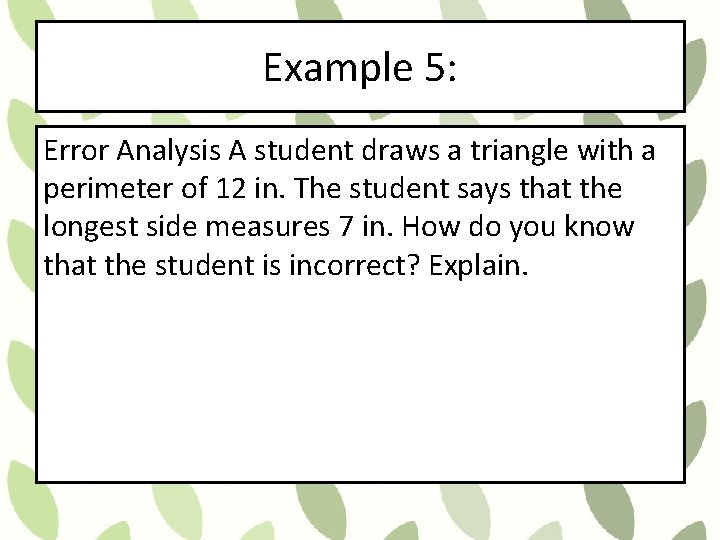Example 5: Error Analysis A student draws a triangle with a perimeter of 12 in. The student says that the longest side measures 7 in. How do you know that the student is incorrect? Explain.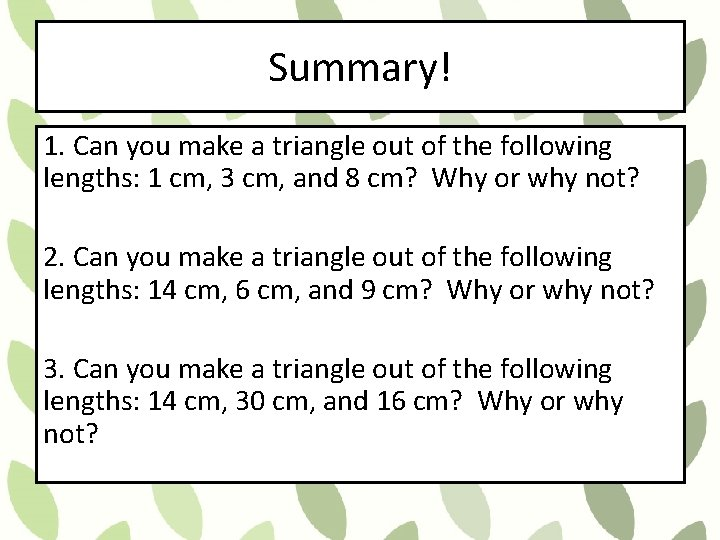Summary! 1. Can you make a triangle out of the following lengths: 1 cm, 3 cm, and 8 cm? Why or why not? 2. Can you make a triangle out of the following lengths: 14 cm, 6 cm, and 9 cm? Why or why not? 3. Can you make a triangle out of the following lengths: 14 cm, 30 cm, and 16 cm? Why or why not?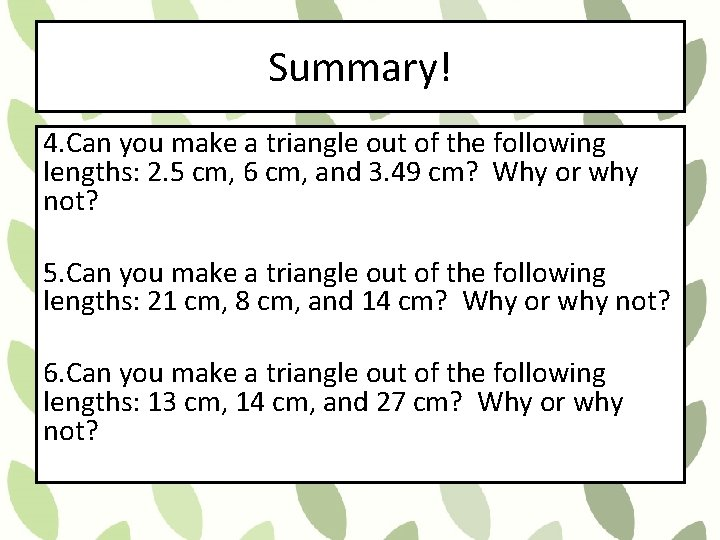Summary! 4. Can you make a triangle out of the following lengths: 2. 5 cm, 6 cm, and 3. 49 cm? Why or why not? 5. Can you make a triangle out of the following lengths: 21 cm, 8 cm, and 14 cm? Why or why not? 6. Can you make a triangle out of the following lengths: 13 cm, 14 cm, and 27 cm? Why or why not?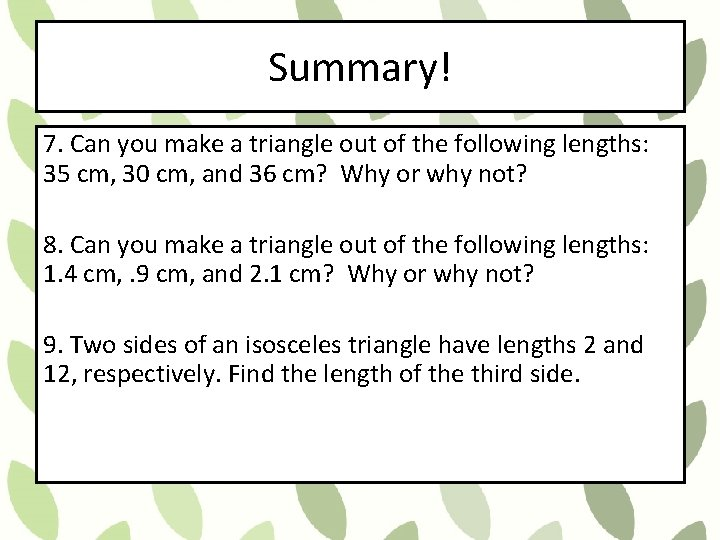Summary! 7. Can you make a triangle out of the following lengths: 35 cm, 30 cm, and 36 cm? Why or why not? 8. Can you make a triangle out of the following lengths: 1. 4 cm, . 9 cm, and 2. 1 cm? Why or why not? 9. Two sides of an isosceles triangle have lengths 2 and 12, respectively. Find the length of the third side.